score
%

Venn Diagrams

Q1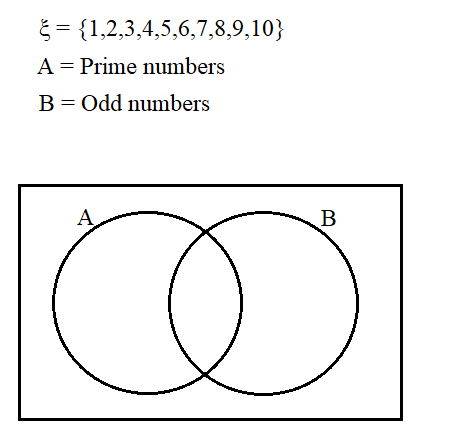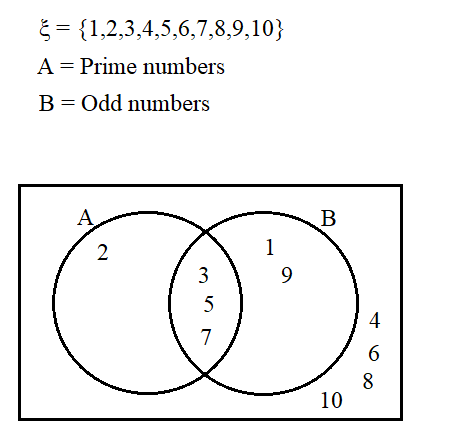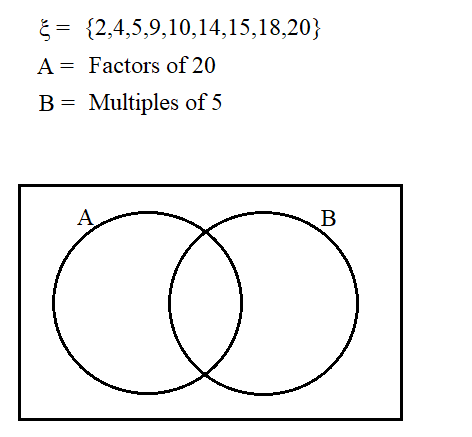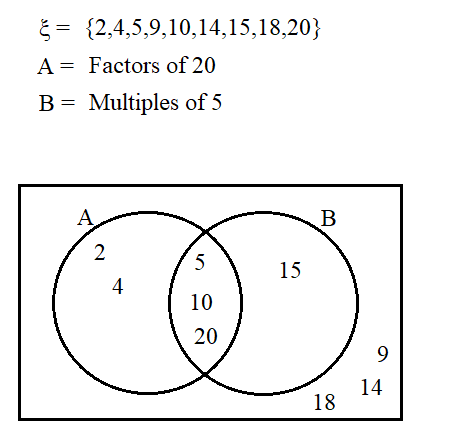a)

Complete the venn diagram

(3 marks)

b)

Find P(A\capB)

(2 marks)

c)

Find P(A\cupB)

(2 marks)

Q2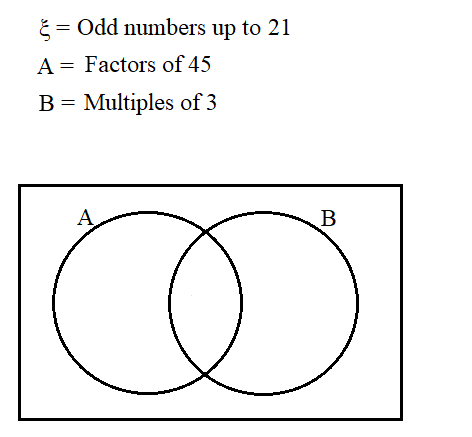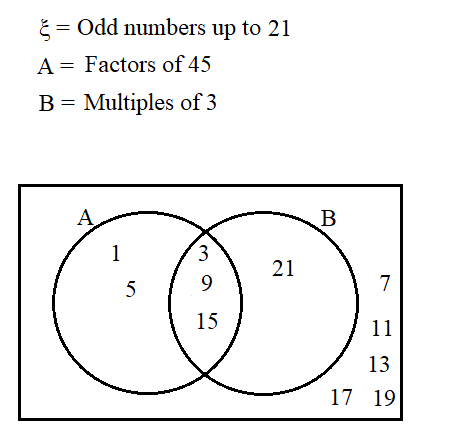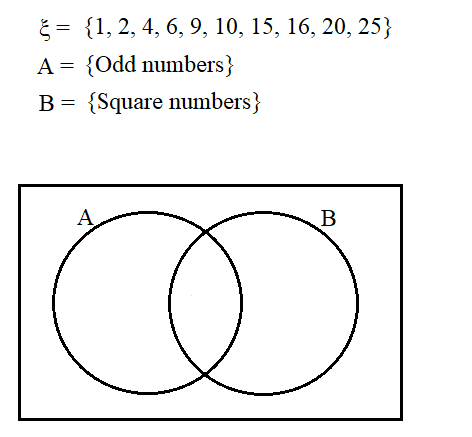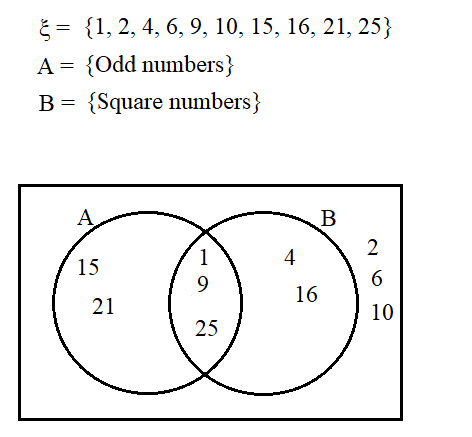a)

Complete the venn diagram

(3 marks)

b)

Find P(A\cupB)

(2 marks)

c)

Find P(A'\capB')

(2 marks)

Q3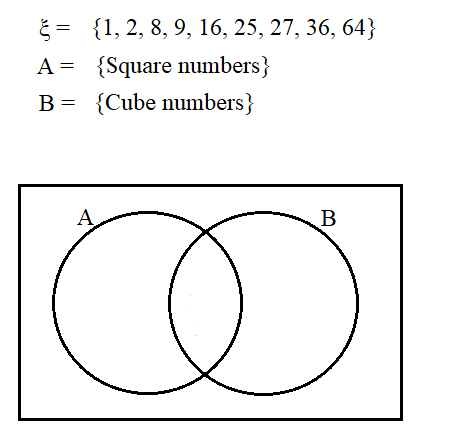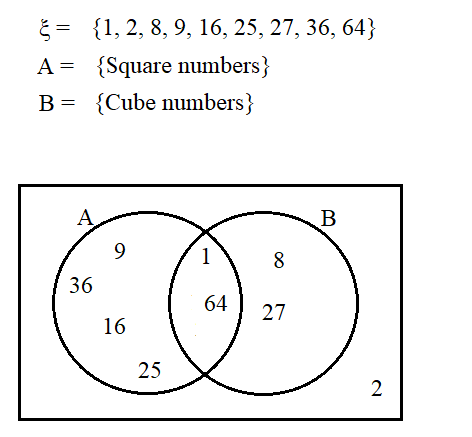a)
Complete the venn diagram
(3 marks)

b)

Find P(A\capB')

(2 marks)

c)

Find P(A'\capB')

(2 marks)

Q4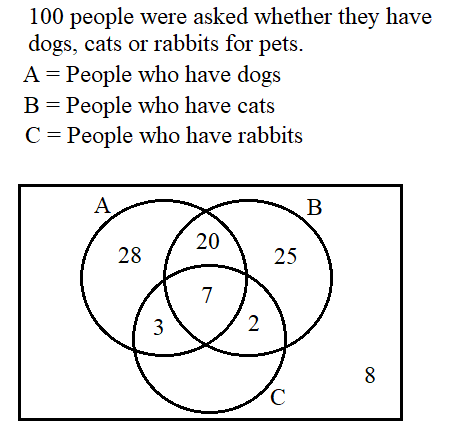a)

How many people only have a rabbit?

(1 mark)

b)

If one of these people were picked at random, what is the probability they would own a cat?

(2 marks)